# 老生常谈比较排序之堆排序

更新时间：2017年06月22日 09:17:35   投稿：jingxian我要评论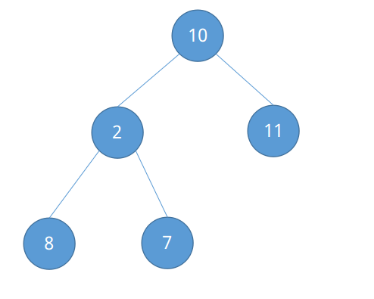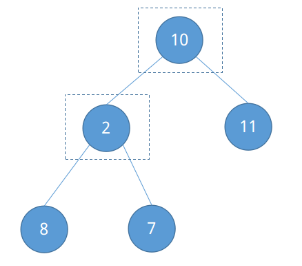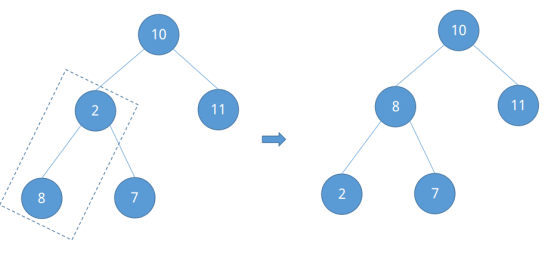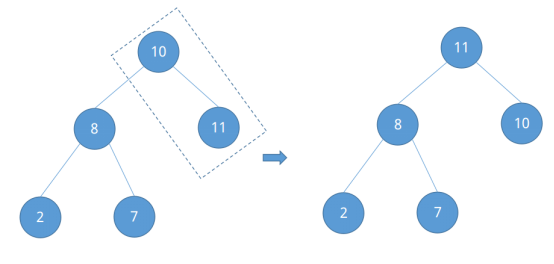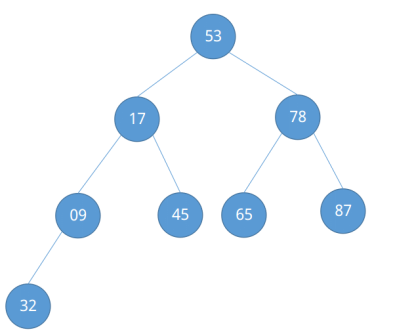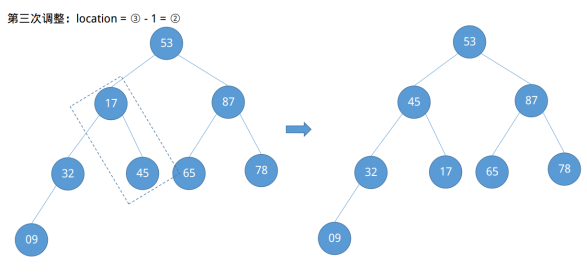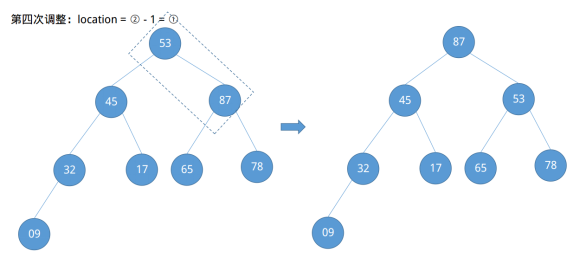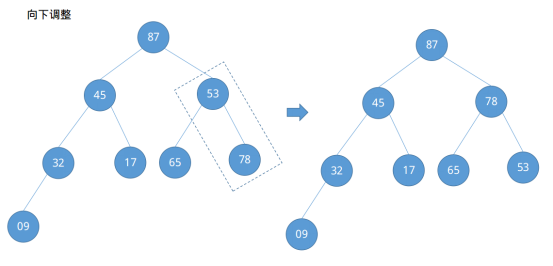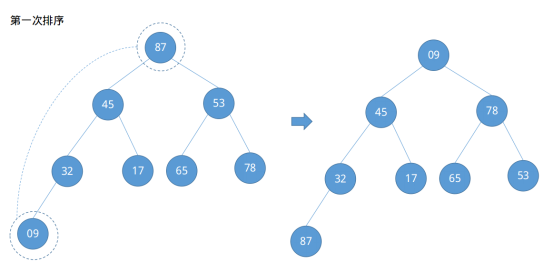Java

```package com.algorithm.sort.heap;

import java.util.Arrays;

/**
* 堆排序
* Created by yulinfeng on 6/20/17.
*/
public class Heap {

public static void main(String[] args) {
int[] nums = {53, 17, 78, 09, 45, 65, 87, 32};
nums = heapSort(nums);
System.out.println(Arrays.toString(nums));
}

/**
* 堆排序
* @param nums 待排序数组序列
* @return 排好序的数组序列
*/
private static int[] heapSort(int[] nums) {

for (int i = nums.length / 2 - 1; i >= 0; i--) {
heapAdjust(nums, i, nums.length);
}
for (int i = nums.length - 1; i > 0; i--) {
int temp = nums[i];
nums[i] = nums;
nums = temp;
heapAdjust(nums, 0, i);
}
return nums;
}

/**
* 调整堆
*
* @param nums  待排序序列
* @param parent   待调整根节点
* @param length 数组序列长度
*/
private static void heapAdjust(int[] nums, int parent, int length) {
int temp = nums[parent];
int childIndex = 2 * parent + 1;  //完全二叉树节点i从编号1开始的左子节点位置在2i，此处数组下标从0开始，即左子节点所在数组索引位置为：2i + 1
while (childIndex < length) {
if (childIndex + 1 < length && nums[childIndex] < nums[childIndex + 1]) {
childIndex++;  //节点有右子节点，且右子节点大于左子节点，则选取右子节点
}
if (temp > nums[childIndex]) {
break; //如果选中节点大于其子节点，直接返回
}
nums[parent] = nums[childIndex];
parent = childIndex;
childIndex = 2 * parent + 1;  //继续向下调整
}
nums[parent] = temp;
}
}```

Python3

```#堆排序
def heap_sort(nums):

for i in range(int(len(nums) / 2 - 1), -1, -1):
heap_adjust(nums, i, len(nums))

for i in range(len(nums) - 1, -1, -1):
temp = nums[i]
nums[i] = nums
nums = temp
heap_adjust(nums, 0, i)

return nums

#调整堆
def heap_adjust(nums, parent, length):

temp = nums[parent]
childIndex = 2 * parent + 1
while childIndex < length:
if childIndex + 1 < length and nums[childIndex] < nums[childIndex + 1]:
childIndex += 1
if temp > nums[childIndex]:
break
nums[parent] = nums[childIndex]
parent = childIndex
childIndex = 2 * parent + 1

nums[parent] = temp

nums = [53, 17, 78, 09, 45, 65, 87, 32]
nums = heap_sort(nums)
print(nums)```